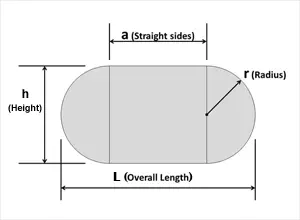If you're planning to build a small stadium for your kids or you're a construction company owner who has signed a contract to build a stadium for 2-3 thousand people, you may find our Stadium Calculator useful. This calculator is designed to help you calculate the area and perimeter of a stadium shape.

A stadium is made up of two-dimensional geometrical shapes, namely a rectangle and a semicircle (also known as a disco rectangle). To use the calculator correctly, please read the following rules and explanations.

### The Stadium Calculator includes the following algorithms:

Height = 2 × r

Overall length = 2 × r + a

Area of a stadium (A) = π × r2 + 2 × r × a

Perimeter of a stadium (p) = 2 × (πr + a)

Where: r is a radius, a is a straight side, π = 3.141592654 to 9 decimal places.